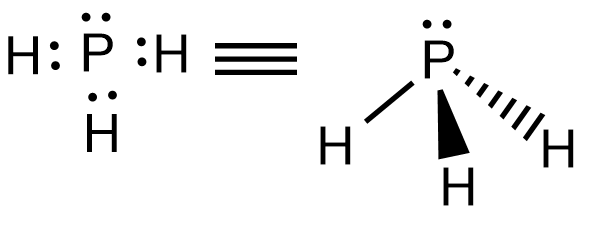# How can I draw the Lewis structure for $P{{H}_{3}}$Verified
175.8k+ views
Hint: Phosphorus belongs to the group 15 and they possess five valence electrons. H belongs to group 1 and has one valence electron.

So in the question it is asked how can we draw a Lewis structure for $P{{H}_{3}}$ molecule. The $P{{H}_{3}}$ is called phosphine and has its IUPAC name as phosphane.
We have to draw the Lewis structure of phosphine, first let’s understand what Lewis structure is and what it tells us about the molecule and the steps to attain the final structure.
Lewis structure is also called as the electron dot structure or electron dot structures, Lewis electron diagram or Lewis electron dot formula.
In Lewis structure we represent the bonds between the atoms as electrons dots. We generally draw the Lewis structures for covalently bonded molecules and also for coordinate compounds.
The Lewis structures gives the possible bonds between the atoms and also tells us if there are lone pairs available for an atom etc.
Now let’s discuss the steps involved in drawing a Lewis dot structure. There are mainly five steps involved, let's check out one by one.
-So the first one is to identify the atoms and calculate the valence electrons of each atom and add up the valence electrons of all the atoms present to get the total valence electrons present in the compound.
-The next step is to find the least electronegative atom in the molecule and we take it as the central atom. For finding which one is least electronegativity we have set a parameter that the atoms away from the F are least electronegative i.e. for example if in a compound like $N{{O}_{2}}$, N is far apart from the F than the O so, N will be taken as the central atom. The same is applicable while down the group. In $PC{{l}_{3}}$, P will be the least electronegative atom than the Cl.
-Next step is to put two electrons between two atoms to show that they are chemically bonded, a minimum of two electrons should be present to form a bond. So first put two electrons between the two atoms.
-Then complete the octet configuration in the outside atoms i.e. the atoms that are attached to the central atom.
-If in the case that the central atom does not have enough electrons to form octet configuration then move a pair of electrons from the outer atom to form the multiple bonds i.e. double or triple bonds between the central atom and the outer atom.
Example -${{O}_{2}}$ molecule.
Now let’s solve and draw the Lewis structure for $P{{H}_{3}}$ by following the steps mentioned above.
-The P possesses 5 valence electrons since it belongs to group -15 and H possesses 1 valence electron as it is placed with the first group elements.
So the total valence electrons present in $P{{H}_{3}}$is,
$Valence\,electrons\,in\,P{{H}_{3}}=5+\left( 3 \right)\times 1=8$
-The P is taken as the central atom to draw the Lewis structure of $P{{H}_{3}}$
Now let’s draw the structure with respect to the remaining three steps:P forms three covalent bonds with three H atoms and P possess a lone pair. Hence the structure of $P{{H}_{3}}$ is trigonal pyramidal.

Note:
While drawing the Lewis structure, H should be always placed as the outside atom, it should always go on outside. That is why H being the least electronegative atom in $P{{H}_{3}}$, P is taken as the central atom.